# 8.8 Vectors  (Page 7/22)

 Page 7 / 22

## Verbal

What are the characteristics of the letters that are commonly used to represent vectors?

lowercase, bold letter, usually $\text{\hspace{0.17em}}u,v,w$

How is a vector more specific than a line segment?

What are $\text{\hspace{0.17em}}i\text{\hspace{0.17em}}$ and $\text{\hspace{0.17em}}j,$ and what do they represent?

They are unit vectors. They are used to represent the horizontal and vertical components of a vector. They each have a magnitude of 1.

What is component form?

When a unit vector is expressed as $⟨a,b⟩,$ which letter is the coefficient of the $\text{\hspace{0.17em}}i\text{\hspace{0.17em}}$ and which the $\text{\hspace{0.17em}}j?$

The first number always represents the coefficient of the $\text{\hspace{0.17em}}i,\text{\hspace{0.17em}}$ and the second represents the $\text{\hspace{0.17em}}j.$

## Algebraic

Given a vector with initial point $\text{\hspace{0.17em}}\left(5,2\right)\text{\hspace{0.17em}}$ and terminal point $\text{\hspace{0.17em}}\left(-1,-3\right),\text{\hspace{0.17em}}$ find an equivalent vector whose initial point is $\text{\hspace{0.17em}}\left(0,0\right).\text{\hspace{0.17em}}$ Write the vector in component form $⟨a,b⟩.$

Given a vector with initial point $\text{\hspace{0.17em}}\left(-4,2\right)\text{\hspace{0.17em}}$ and terminal point $\text{\hspace{0.17em}}\left(3,-3\right),\text{\hspace{0.17em}}$ find an equivalent vector whose initial point is $\text{\hspace{0.17em}}\left(0,0\right).\text{\hspace{0.17em}}$ Write the vector in component form $⟨a,b⟩.$

$〈7,-5〉$

Given a vector with initial point $\text{\hspace{0.17em}}\left(7,-1\right)\text{\hspace{0.17em}}$ and terminal point $\text{\hspace{0.17em}}\left(-1,-7\right),\text{\hspace{0.17em}}$ find an equivalent vector whose initial point is $\text{\hspace{0.17em}}\left(0,0\right).\text{\hspace{0.17em}}$ Write the vector in component form $⟨a,b⟩.$

For the following exercises, determine whether the two vectors $\text{\hspace{0.17em}}u\text{\hspace{0.17em}}$ and $\text{\hspace{0.17em}}v\text{\hspace{0.17em}}$ are equal, where $\text{\hspace{0.17em}}u\text{\hspace{0.17em}}$ has an initial point $\text{\hspace{0.17em}}{P}_{1}\text{\hspace{0.17em}}$ and a terminal point $\text{\hspace{0.17em}}{P}_{2}\text{\hspace{0.17em}}$ and $v$ has an initial point $\text{\hspace{0.17em}}{P}_{3}\text{\hspace{0.17em}}$ and a terminal point $\text{\hspace{0.17em}}{P}_{4}$ .

${P}_{1}=\left(5,1\right),{P}_{2}=\left(3,-2\right),{P}_{3}=\left(-1,3\right),\text{\hspace{0.17em}}$ and $\text{\hspace{0.17em}}{P}_{4}=\left(9,-4\right)$

not equal

${P}_{1}=\left(2,-3\right),{P}_{2}=\left(5,1\right),{P}_{3}=\left(6,-1\right),\text{\hspace{0.17em}}$ and $\text{\hspace{0.17em}}{P}_{4}=\left(9,3\right)$

${P}_{1}=\left(-1,-1\right),{P}_{2}=\left(-4,5\right),{P}_{3}=\left(-10,6\right),\text{\hspace{0.17em}}$ and $\text{\hspace{0.17em}}{P}_{4}=\left(-13,12\right)$

equal

${P}_{1}=\left(3,7\right),{P}_{2}=\left(2,1\right),{P}_{3}=\left(1,2\right),\text{\hspace{0.17em}}$ and $\text{\hspace{0.17em}}{P}_{4}=\left(-1,-4\right)$

${P}_{1}=\left(8,3\right),{P}_{2}=\left(6,5\right),{P}_{3}=\left(11,8\right),\text{\hspace{0.17em}}$ and ${P}_{4}=\left(9,10\right)$

equal

Given initial point $\text{\hspace{0.17em}}{P}_{1}=\left(-3,1\right)\text{\hspace{0.17em}}$ and terminal point $\text{\hspace{0.17em}}{P}_{2}=\left(5,2\right),\text{\hspace{0.17em}}$ write the vector $\text{\hspace{0.17em}}v\text{\hspace{0.17em}}$ in terms of $\text{\hspace{0.17em}}i\text{\hspace{0.17em}}$ and $\text{\hspace{0.17em}}j.\text{\hspace{0.17em}}$

Given initial point $\text{\hspace{0.17em}}{P}_{1}=\left(6,0\right)\text{\hspace{0.17em}}$ and terminal point $\text{\hspace{0.17em}}{P}_{2}=\left(-1,-3\right),\text{\hspace{0.17em}}$ write the vector $\text{\hspace{0.17em}}v\text{\hspace{0.17em}}$ in terms of $\text{\hspace{0.17em}}i\text{\hspace{0.17em}}$ and $\text{\hspace{0.17em}}j.\text{\hspace{0.17em}}$

$7i-3j$

For the following exercises, use the vectors u = i + 5 j , v = −2 i − 3 j ,  and w = 4 i j .

Find u + ( v w )

Find 4 v + 2 u

$-6i-2j$

For the following exercises, use the given vectors to compute u + v , u v , and 2 u − 3 v .

$u=⟨2,-3⟩,v=⟨1,5⟩$

$u=⟨-3,4⟩,v=⟨-2,1⟩$

$u+v=〈-5,5〉,u-v=〈-1,3〉,2u-3v=〈0,5〉$

Let v = −4 i + 3 j . Find a vector that is half the length and points in the same direction as $\text{\hspace{0.17em}}v.$

Let v = 5 i + 2 j . Find a vector that is twice the length and points in the opposite direction as $\text{\hspace{0.17em}}v.$

$-10i–4j$

For the following exercises, find a unit vector in the same direction as the given vector.

a = 3 i + 4 j

b = −2 i + 5 j

$-\frac{2\sqrt{29}}{29}i+\frac{5\sqrt{29}}{29}j$

c = 10 i j

$d=-\frac{1}{3}i+\frac{5}{2}j$

$-\frac{2\sqrt{229}}{229}i+\frac{15\sqrt{229}}{229}j$

u = 100 i + 200 j

u = −14 i + 2 j

$-\frac{7\sqrt{2}}{10}i+\frac{\sqrt{2}}{10}j$

For the following exercises, find the magnitude and direction of the vector, $\text{\hspace{0.17em}}0\le \theta <2\pi .$

$⟨0,4⟩$

$⟨6,5⟩$

$|v|=7.810,\theta =39.806°$

$⟨2,-5⟩$

$⟨-4,-6⟩$

$|v|=7.211,\theta =236.310°$

Given u = 3 i − 4 j and v = −2 i + 3 j , calculate $\text{\hspace{0.17em}}u\cdot v.$

Given u = − i j and v = i + 5 j , calculate $\text{\hspace{0.17em}}u\cdot v.$

$-6$

Given $\text{\hspace{0.17em}}u=⟨-2,4⟩\text{\hspace{0.17em}}$ and $\text{\hspace{0.17em}}v=⟨-3,1⟩,\text{\hspace{0.17em}}$ calculate $\text{\hspace{0.17em}}u\cdot v.$

Given u $=⟨-1,6⟩$ and v $=⟨6,-1⟩,$ calculate $\text{\hspace{0.17em}}u\cdot v.$

$-12$

## Graphical

For the following exercises, given $\text{\hspace{0.17em}}v,\text{\hspace{0.17em}}$ draw $v,$ 3 v and $\text{\hspace{0.17em}}\frac{1}{2}v.$

$⟨2,-1⟩$

$⟨-1,4⟩$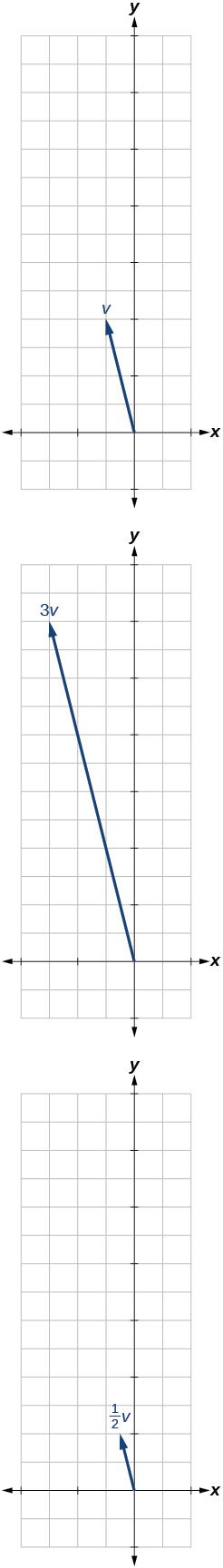$⟨-3,-2⟩$

For the following exercises, use the vectors shown to sketch u + v , u v , and 2 u .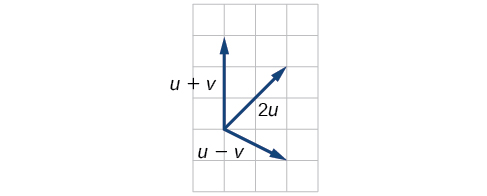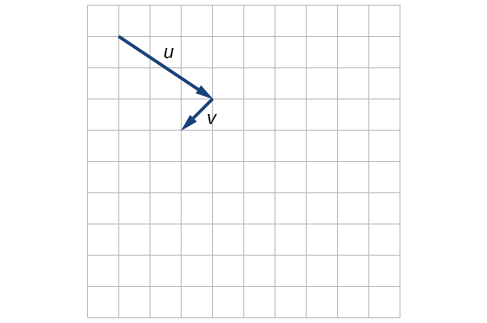For the following exercises, use the vectors shown to sketch 2 u + v .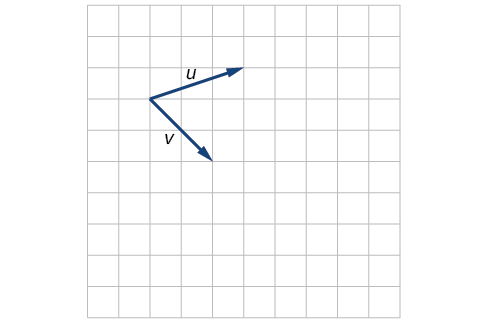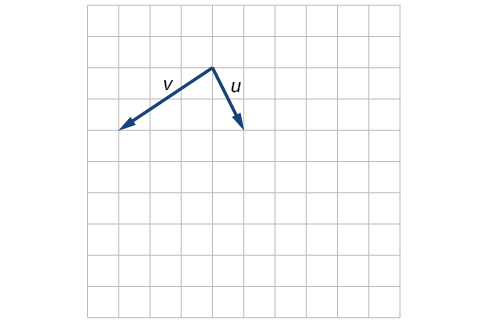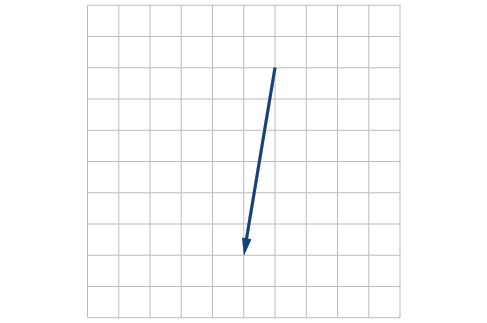For the following exercises, use the vectors shown to sketch u − 3 v .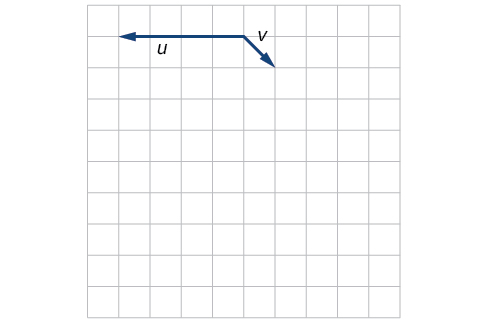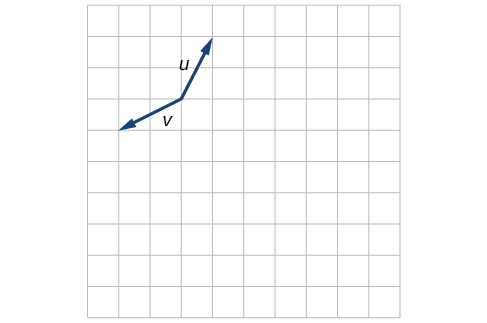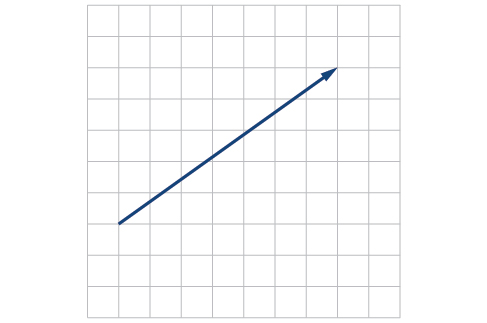For the following exercises, write the vector shown in component form.

give me an example of a problem so that I can practice answering
x³+y³+z³=42
Robert
dont forget the cube in each variable ;)
Robert
of she solves that, well ... then she has a lot of computational force under her command ....
Walter
what is a function?
I want to learn about the law of exponent
explain this
what is functions?
A mathematical relation such that every input has only one out.
Spiro
yes..it is a relationo of orders pairs of sets one or more input that leads to a exactly one output.
Mubita
Is a rule that assigns to each element X in a set A exactly one element, called F(x), in a set B.
RichieRich
If the plane intersects the cone (either above or below) horizontally, what figure will be created?
can you not take the square root of a negative number
No because a negative times a negative is a positive. No matter what you do you can never multiply the same number by itself and end with a negative
lurverkitten
Actually you can. you get what's called an Imaginary number denoted by i which is represented on the complex plane. The reply above would be correct if we were still confined to the "real" number line.
Liam
Suppose P= {-3,1,3} Q={-3,-2-1} and R= {-2,2,3}.what is the intersection
can I get some pretty basic questions
In what way does set notation relate to function notation
Ama
is precalculus needed to take caculus
It depends on what you already know. Just test yourself with some precalculus questions. If you find them easy, you're good to go.
Spiro
the solution doesn't seem right for this problem
what is the domain of f(x)=x-4/x^2-2x-15 then
x is different from -5&3
Seid
All real x except 5 and - 3
Spiro
***youtu.be/ESxOXfh2Poc
Loree
how to prroved cos⁴x-sin⁴x= cos²x-sin²x are equal
Don't think that you can.
Elliott
By using some imaginary no.
Tanmay
how do you provided cos⁴x-sin⁴x = cos²x-sin²x are equal
What are the question marks for?
Elliott
Someone should please solve it for me Add 2over ×+3 +y-4 over 5 simplify (×+a)with square root of two -×root 2 all over a multiply 1over ×-y{(×-y)(×+y)} over ×y
For the first question, I got (3y-2)/15 Second one, I got Root 2 Third one, I got 1/(y to the fourth power) I dont if it's right cause I can barely understand the question.
Is under distribute property, inverse function, algebra and addition and multiplication function; so is a combined question
AbenaByByByBy OpenStaxBy OpenStaxBy Richley CrapoBy JavaChamp TeamBy Joli JuliannaBy JavaChamp TeamBy OpenStaxBy Cath YuBy Jordon Humphreys